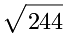# Using the Pythagorean Theorem - Set 2

### Explanation:

A right triangle has a right angle.  A man named Pythagoras recognized there is a special relationship between the lengths of the sides of the triangle.  That relationship is shown by the formula c² = a² + b² and is known as the Pythagorean Theorem.  The "a" and "b" are the lengths of the two sides that form the right angle.  Those two sides are known as the "legs".  The "c" is the length of the third side and is known as the "hypotenuse".

### Example(s):

A right triangle may have legs that have lengths of 10 & 12.  Using the Pythagorean Theorem, the c² = a² + b² becomes c² = 10² + 12² which becomes c² = 100 + 144 which becomes c² = 244.  Now you can list the square monumentalmath of each side and the equation becomes c =.

### Directions:

For each problem in this problem set you will be given the lengths of the legs, such as 3 & 11.  Use the Pythagorean Theorem to find the length of the hypotenuse.  Strive for ease of using the Pythagorean Theorem and then strive for speed.  Good luck and enjoy the challenge!

...